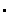# Appended Note 2-4 Estimation method of expenditures and the value of support

[Toc]  [Prev]  [Next]

## 1. Estimation method of the value of support

The remaining amounts of loans provided in the past by each fiscal year were estimated in connection to the outstanding loans at the end of each fiscal year based on the amount of new loans provided by the policy-based finance institutions each fiscal year. The remaining amounts of loans were multiplied to the power of the estimated interest of each fiscal year and these values were added together. Then the revenue from interest charge for each fiscal year when a private financial institution provides loans of a similar amount was estimated from the sum derived above.

The estimated interest has been calculated while the gap between the periods of loan has been corrected through utilizing the contracted interest for new loans, which is the average interest that is charged on new loans provided by private financial institutions. Such measures were taken given the fact that the periods of loan for those provided by policy-based finance institutions are longer than those by the private finance institutions. While assuming that the average period of loans provided by the private financial institutions is two years, the gap between this and the average period of new loans provided by policy-based finance institutions was corrected, based on the distribution yield of government bonds at the end of each fiscal year.

By following the above procedures, the gap between the revenue from the interest rate on loans given in the income statement of the policy-based finance institutions, and the revenue from the estimated interest discussed above for each fiscal year has been calculated and determined as the value of support.

## 2. Estimation method of expenditure

This has been defined as the fiscal burden of the government for each fiscal year (the opportunity cost and subsidy related to the capital invested by the policy-based finance institutions). The opportunity cost has been calculated by multiplying the amount of capital invested in each fiscal year to the power of the distribution yield of government bonds.

## 3. Estimation method by institutions

(1) Japan Bank for International Cooperation (JBIC)The average period of new loans was set at 11 years in connection to the calculation of the estimated interest.Only includes the yen-denominated loans of the International Finance Operation (former Export-Import Bank of Japan (EIB)).

(2) Development Bank of JapanThe average period of new loans was set at 14 years in connection to the calculation of the estimated interest.

(3) Okinawa Development Finance CorporationIn calculating the estimated interest, the gap between the distribution yield of government bonds at the end of each fiscal year equivalent to the gap between the period of new loans (Okinawa Development Finance Corporation 20 years; private financial institutions 2 years) was added to the contracted interest for new loans (long-term). As for housing loans, however, the gap between the distribution yield of government bonds at the end of each fiscal year equivalent to the gap between the period of new loans (Okinawa Development Finance Corporation 30 years; private financial institutions 10 years) was added to the interest for housing loan (fixed) of private financial institutions.

(4) Japan Finance Corporation for Municipal EnterprisesThe average period of new loans was set at 24 years in connection to the calculation of the estimated interest.

(5) National Life Finance CorporationThe average period of new loans was set at seven years in connection to the calculation of the estimated interest.

(6) Agriculture, Forestry and Fisheries Finance CorporationThe average period of new loans was set at 18 years in connection to the calculation of the estimated interest.

(7) Japan Finance Corporation for Small and Medium EnterpriseThe average period of new loans was set at nine years in connection to the calculation of the estimated interest.

(8) Government Housing Loan CorporationIn calculating the estimated interest, the gap between the distribution yield of government bonds at the end of each fiscal year equivalent to the gap between the period of new loans (Government Housing Loan Corporation 30 years; private financial institutions 10 years) was added to the interest for housing loan (fixed) of private financial institutions.

(9) Shoko Chukin BankIn calculating the estimated interest for long term loans, the gap between the distribution yield of government bonds at the end of each fiscal year equivalent to the gap between the period of new loans (Shoko Chukin Bank 5 years; private financial institutions 2 years) was added to the contracted interest for new loans (long-term). As for short term loans, the contracted interest for new loans (short term) was used.

## 4. Expenditure to value of support ratio

(1) Expenditure to value of support ratio (times) = value of support/government financial burden

(2) Value of support (million yen) per unit loan (100 million yen)=value of support/average outstanding loans during the term

(3) Net value of support (million yen) per unit loan (100 million yen)=(value of support - government financial burden)/ average outstanding loans during the term

Source: Policy Evaluation Report on the Supply of Public Funds by Policy-Based Finance Institutions, etc., Ministry of Internal Affairs and Communications; Policy (Subsidy) Cost Analysis of Fiscal Investment and Loan Program Projects, Ministry of Finance; Economic Statistics Annual, Bank of Japan and materials on the settlement and IR of each institution.

[Toc]  [Prev]  [Next]